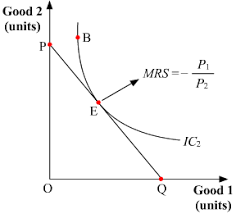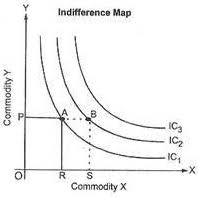# Preferences of the Consumer: Consumer Preference, Marginal Utility etc.

The compilation of these Consumer Behaviour Notes makes students exam preparation simpler and organised.

## Preferences of the Consumer

Consumer preference is crucial to Microeconomics. Concepts such as utility, budget line, indifference curve, and indifference map sound complex at once but are easy to understand as can be. Let us understand Consumer Preference Theory to understand consumer behaviour and how consumers make choices.

### Consumer Preference Theory

To understand consumer behaviour, it is important to know what guides consumer preferences. Central to consumer preferences is the idea of utility. What is meant by ‘utility’?

Utility refers to the ability of a commodity to serve human wants. It is the amount of satisfaction a consumer gets from the consumption of a good or service. ‘Utility’ can be of two types:

Cardinal Utility Approach

• Also known as Marginal Utility Analysis.
• Cardinal utility theory states that utility is measurable in number.
• The unit in which utility can be measured is ‘utils’.
• Thus, those goods that give a consumer a higher level of satisfaction will be assigned higher utils than those which give the consumer lower satisfaction.
• Cardinal theory is a quantitative method of utility measurement.

Ordinal Utility Approach

• Also known as Indifference Curve Analysis.
• It states that satisfaction derivable from the consumption of goods cannot be measured in numbers.
• It uses ‘ranks’ to describe different levels of utility.
• Thus, goods that provide a higher level of satisfaction should be assigned higher ranks than those which give the consumer lower satisfaction.
• The ordinal theory is a qualitative method of utility measurement.

### Important Terminologies

Marginal Utility: It is the additional satisfaction obtained from consuming one more unit of a commodity. It is the change in total utility brought about by one more unit consumption of the commodity.

Total Utility: It is the total psychological satisfaction obtained from consuming a given amount of a commodity. Thus, it is the sum of all marginal utilities obtained from consuming each successive unit of a commodity.

Both, marginal and total utilities are measured in ‘utils’. Thus, TU = ∑ MU. And, MU = ΔTU / ΔQ can be derived from the above two definitions. You must note that since MU is the change in TU from consuming one more unit, it can also be written as:

MU = TUn – TUn-1

This will become simple with the table below. If the commodity is an orange, MU of 2 units of orange using the first definition of MU is, using the second definition of MU, MU of 2 units is again, 18 – 10 = 8. To calculate TU, we perform cumulative addition over MU. So, the TU of 2 units of orange is 10 + 8 = 18. Thus, if one of MU or TU is given, the other can be found out easily.### Law of Diminishing Marginal Utility

The crucial point of consumer preference theory is this law. It states that as more and more of a commodity is consumed, consumers receive less and less satisfaction from its consumption. More formally, it means that the Marginal utility of a commodity declines as successive units of it are consumed. A corollary of this law is that the Total utility of the commodity increases at a diminishing rate (see table above).

To understand the relationship between MU and TU, note that:

When MU decreases but remains positive, TU increases at a diminishing rate.
When MU = 0, TU reaches its maximum.
Also when MU is negative, TU starts declining.### Indifference Curve Analysis

We have seen bundles that give more satisfaction to a consumer are ‘preferred’ to other bundles. But what happens when some bundles give the same level of satisfaction to a consumer? The consumer becomes indifferent between such bundles and does not prefer one over another. A diagrammatic representation of all such bundles between which the consumer is indifferent is called an indifference curve. It depicts various combinations of two goods that give a consumer the same level of satisfaction. A collection of indifference curves is called an indifference map.Properties of Indifference Curve

• An indifference curve is downward sloping.
• An indifference curve is convex to the origin.
• No two indifference curves can intersect each other.
• A higher indifference curve depicts a higher level of satisfaction.

Optimum Combination
The condition for consumer’s optimum choice of two goods can be explained with the help of the Indifference Curve and Budget Line. An indifference curve indicates satisfaction, while the budget line indicates affordability. A consumer would want to maximize his satisfaction from two goods subject to his budget constraint. Thus, he will want to reach the highest possible IC which falls within his budget. This can happen when:

The budget line is tangent to the IC.
Slope of IC = Slope of Budget Line (MRS = -)Example:

Question:
Why does the Indifference Curve have a negative slope?